# DAV Class 3 Maths Chapter 6 Worksheet 3 Solutions

The  DAV Class 3 Maths Solutions and DAV Class 3 Maths Chapter 6 Worksheet 3 Solutions of Day, Date and Time offer comprehensive answers to textbook questions.

## DAV Class 3 Maths Ch 6 WS 3 Solutions

Question 1.
Fill in the blanks. The first question is done for you.

(a) The hour hand is between 6 and 7. The minute hand is at 8.Solution:
Time: 40 minutes past 6 or 6 : 40.

(b) The hour hand is between ____ and ____
The minute hand is at ____ .
Time: ____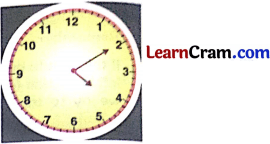Solution:
4 and 5 (hour hand), 2 (minute hand),
Time: Ten minutes past 4 or 4 : 10.(c) The hour hand is between ____ and ____
The minute hand is at ____
Time: ____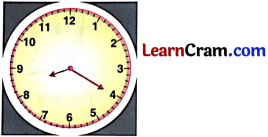Solution:
8 and 9 (hour hand), 4 (minute hand).
Time: Twenty minutes past Eight or 8:20.

(d) The hour hand is between ____ and ____
The minute hand is at ____
Time: ____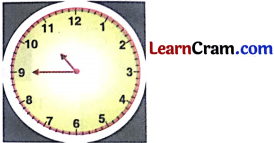Solution:
10 and 11 (hour hand), 9 (minutes hand)
Time : Quarter to 11. or 10 : 45.Question 2.
Write the following times in numbers. The first one is done for you.

(a) Half past 4 ____
Solution:
4 : 30

(b) Quarter past 9 ____
Solution:
9 : 15

(c) 20 minutes past 6 ____
Solution:
6 : 20

(d) 10 minutes to 3 ____
Solution:
2 : 50

(e) Quarter to 2 ____
Solution:
1 : 45

(f) 15 minutes past 7 ____
Solution:
7 : 15Question 3.
Write the following times in words.

(a) 1 : 15 = ________
Solution:
15 minutes past 1

(b) 4 : 35 = ________
Solution:
35 minutes past 4

(c) 11 : 10 = ________
Solution:
10 minutes past 11

(d) 12 : 05 = ________
Solution:
5 minutes past 12Question 4.
What time does the clock show? Write in words and numbers.

(a)Solution:

5 minutes past 7
7 : 05

(b)Solution:

quarter to 4
3 : 45

(c)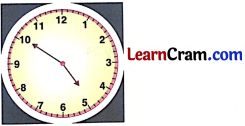Solution:
10 minutes to 5
4 : 50(d)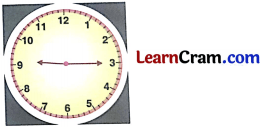Solution:
quarter past 9
9 : 15

(e)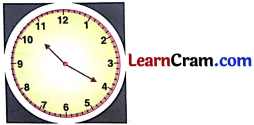Solution:
20 minutes past 10
10 : 20

(f)Solution:
10 minutes past 5
5 : 10### DAV Class 3 Maths Chapter 6 Worksheet 3 Notes

Finding Time in $$\frac{1}{4}$$ hours and in Minutes

If the minute hand is on the left hand side (6 to 12), we say ‘to’ the hour.
If the minute hand is on the right hand side (12 to 6), we say ‘past’ the hour.

Example: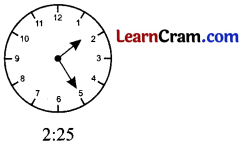The minute hand is at right hand side.
Time : 25 minutes past 2The minute hand is at left hand side.
Time : 10 minutes past 3Time 11 : 15
We say Quarter past 11. The hour hand has left the mark of 11.
Time 10 : 45
We say quarter to 11. The hour hand is nearing the mark of 11.